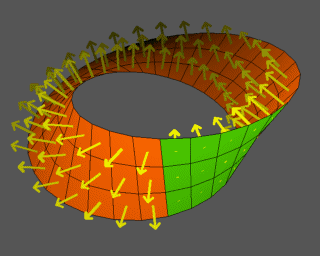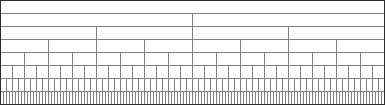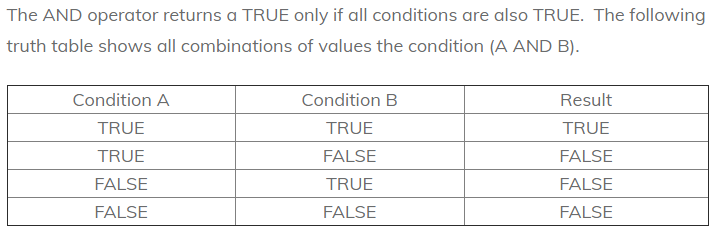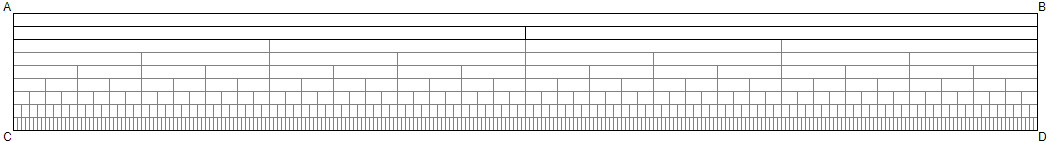Closed Loop Interval Ontology
 CLOSED LOOP INTERVAL ONTOLOGY        The Digital Integration of Conceptual FormORIGINPlease sign in or register Email * Password * Searches selected display Project under development Evolving and coalescing Guiding motivation Why we do this Objectives and strategy Reconciliation and integration Reconciliation of perspectives Holistic view on alternatives What is truth? How do we know? What is a concept? Definitions and alternatives Semantics How meaning is created Universal Hierarchy Spectrum of levels A Universal Foundation The closed loop ensemble contains all primary definitions Set Dimensions of set theory Venn diagrams Topology of sets Objects in Boolean Algebra How are they constructed? Core vocabulary Primary terms Core terms on the strip Closed Loop framework Graphics Hierarchical models Digital geometry Euclid in digital space The digital integration of conceptual form Compositional semantics Closed loop interval ontology How it works Cognitive science The integrated science of mind Equality What does it mean? Formal systematic definitions Core terms Data structures Constructive elements and building blocks Compactification Preserving data under transformation Steady-state cosmology In the beginning Semantic ontology Domain and universal Articles From other sourcesObjects in Boolean Algebra
How are they constructed?

 In mathematics and mathematical logic, Boolean algebra is the branch of algebra in which the values of the variables are the truth values true and false, usually denoted 1 and 0, respectively. Instead of elementary algebra, where the values of the variables are numbers and the prime operations are addition and multiplication, the main operations of Boolean algebra are the conjunction (and) denoted as ?, the disjunction (or) denoted as ?, and the negation (not) denoted as ¬. It is thus a formalism for describing logical operations, in the same way that elementary algebra describes numerical operations. Boolean algebra was introduced by George Boole in his first book The Mathematical Analysis of Logic (1847), and set forth more fully in his An Investigation of the Laws of Thought (1854). According to Huntington, the term "Boolean algebra" was first suggested by Sheffer in 1913, although Charles Sanders Peirce gave the title "A Boolean Algebra with One Constant" to the first chapter of his "The Simplest Mathematics" in 1880. Boolean algebra has been fundamental in the development of digital electronics, and is provided for in all modern programming languages. It is also used in set theory and statistics.https://en.wikipedia.org/wiki/Boolean_algebra_(structure)

 Boolean values In Boolean Algebra, the values of the variables are true and false (usually denoted 1 and 0). So, the structure of something that is true or false is some kind of proposition -- or in a programming context, a condition that is true -- is is the case at the moment -- IF x=20, then y -- or something like that Reference In mathematics and mathematical logic, Boolean algebra is the branch of algebra in which the values of the variables are the truth values true and false, usually denoted 1 and 0, respectively. Instead of elementary algebra, where the values of the variables are numbers and the prime operations are addition and multiplication, the main operations of Boolean algebra are the conjunction (and) denoted as ?, the disjunction (or) denoted as ?, and the negation (not) denoted as ¬. It is thus a formalism for describing logical operations, in the same way that elementary algebra describes numerical operations. Boolean algebra was introduced by George Boole in his first book The Mathematical Analysis of Logic (1847), and set forth more fully in his An Investigation of the Laws of Thought (1854). According to Huntington, the term "Boolean algebra" was first suggested by Sheffer in 1913, although Charles Sanders Peirce gave the title "A Boolean Algebra with One Constant" to the first chapter of his "The Simplest Mathematics" in 1880. Boolean algebra has been fundamental in the development of digital electronics, and is provided for in all modern programming languages. It is also used in set theory and statistics.